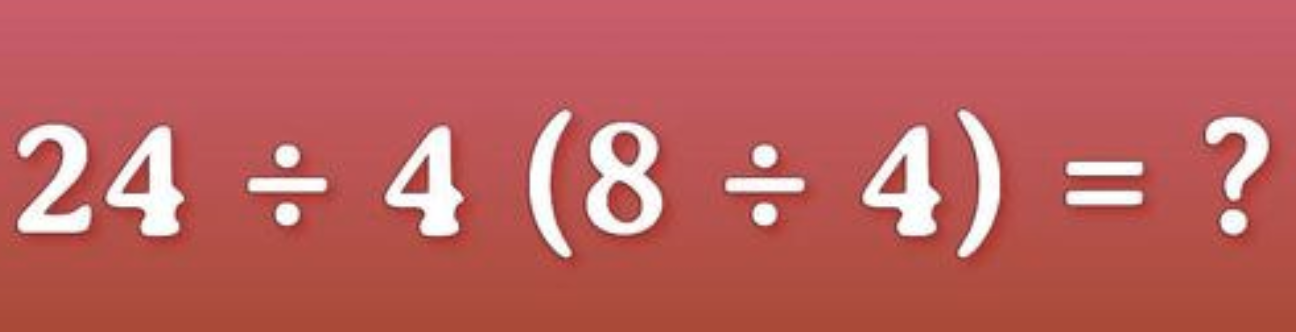You might not use algebra or trigonometry every single day, but you need basic arithmetic to navigate through this world.   It’s good to practice your math skills to keep them sharp.  Now, why don’t you give it a try below?What’s the answer? Do you think it’s or 12?

If you used the PEMDAS rule you would have to do the question in the parentheses first, which is 8 ÷ 4, which equals 2.

Then you must shift over to the left equation and do 24 ÷ 4= 6.

Then you have to multiply 6 x 2 which equals 12 and there is your answer!

So did you get it right?